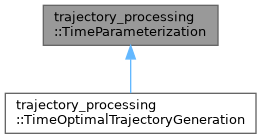moveit2 The MoveIt Motion Planning Framework for ROS 2.
trajectory_processing::TimeParameterization Class Referenceabstract

`#include <time_parameterization.h>`

Inheritance diagram for trajectory_processing::TimeParameterization:[legend]

## Public Member Functions

TimeParameterization ()=default

TimeParameterization (const TimeParameterization &)=default

TimeParameterization (TimeParameterization &&)=default

TimeParameterizationoperator= (const TimeParameterization &)=default

TimeParameterizationoperator= (TimeParameterization &&)=default

virtual ~TimeParameterization ()=default

virtual bool computeTimeStamps (robot_trajectory::RobotTrajectory &trajectory, const double max_velocity_scaling_factor=1.0, const double max_acceleration_scaling_factor=1.0) const =0
Compute a trajectory with waypoints spaced equally in time. More...

virtual bool computeTimeStamps (robot_trajectory::RobotTrajectory &trajectory, const std::unordered_map< std::string, double > &velocity_limits, const std::unordered_map< std::string, double > &acceleration_limits, const double max_velocity_scaling_factor=1.0, const double max_acceleration_scaling_factor=1.0) const =0
Compute a trajectory with waypoints spaced equally in time. More...

virtual bool computeTimeStamps (robot_trajectory::RobotTrajectory &trajectory, const std::vector< moveit_msgs::msg::JointLimits > &joint_limits, const double max_velocity_scaling_factor=1.0, const double max_acceleration_scaling_factor=1.0) const =0
Compute a trajectory with waypoints spaced equally in time. More...

## Detailed Description

Definition at line 11 of file time_parameterization.h.

## ◆ TimeParameterization() [1/3]

 trajectory_processing::TimeParameterization::TimeParameterization ( )
default

## ◆ TimeParameterization() [2/3]

 trajectory_processing::TimeParameterization::TimeParameterization ( const TimeParameterization & )
default

## ◆ TimeParameterization() [3/3]

 trajectory_processing::TimeParameterization::TimeParameterization ( TimeParameterization && )
default

## ◆ ~TimeParameterization()

 virtual trajectory_processing::TimeParameterization::~TimeParameterization ( )
virtualdefault

## ◆ computeTimeStamps() [1/3]

 virtual bool trajectory_processing::TimeParameterization::computeTimeStamps ( robot_trajectory::RobotTrajectory & trajectory, const double max_velocity_scaling_factor = `1.0`, const double max_acceleration_scaling_factor = `1.0` ) const
pure virtual

Compute a trajectory with waypoints spaced equally in time.

Parameters
 [in,out] trajectory A path which needs time-parameterization. It's OK if this path has already been time-parameterized; this function will re-time-parameterize it. max_velocity_scaling_factor A factor in the range [0,1] which can slow down the trajectory. max_acceleration_scaling_factor A factor in the range [0,1] which can slow down the trajectory.

Implemented in trajectory_processing::TimeOptimalTrajectoryGeneration.

## ◆ computeTimeStamps() [2/3]

 virtual bool trajectory_processing::TimeParameterization::computeTimeStamps ( robot_trajectory::RobotTrajectory & trajectory, const std::unordered_map< std::string, double > & velocity_limits, const std::unordered_map< std::string, double > & acceleration_limits, const double max_velocity_scaling_factor = `1.0`, const double max_acceleration_scaling_factor = `1.0` ) const
pure virtual

Compute a trajectory with waypoints spaced equally in time.

Parameters
 [in,out] trajectory A path which needs time-parameterization. It's OK if this path has already been time-parameterized; this function will re-time-parameterize it. velocity_limits Joint names and velocity limits in rad/s acceleration_limits Joint names and acceleration limits in rad/s^2 max_velocity_scaling_factor A factor in the range [0,1] which can slow down the trajectory. max_acceleration_scaling_factor A factor in the range [0,1] which can slow down the trajectory.

Implemented in trajectory_processing::TimeOptimalTrajectoryGeneration.

## ◆ computeTimeStamps() [3/3]

 virtual bool trajectory_processing::TimeParameterization::computeTimeStamps ( robot_trajectory::RobotTrajectory & trajectory, const std::vector< moveit_msgs::msg::JointLimits > & joint_limits, const double max_velocity_scaling_factor = `1.0`, const double max_acceleration_scaling_factor = `1.0` ) const
pure virtual

Compute a trajectory with waypoints spaced equally in time.

Parameters
 [in,out] trajectory A path which needs time-parameterization. It's OK if this path has already been time-parameterized; this function will re-time-parameterize it. joint_limits Joint names and corresponding velocity limits in rad/s and acceleration limits in rad/s^2 max_velocity_scaling_factor A factor in the range [0,1] which can slow down the trajectory. max_acceleration_scaling_factor A factor in the range [0,1] which can slow down the trajectory.

Implemented in trajectory_processing::TimeOptimalTrajectoryGeneration.

## ◆ operator=() [1/2]

 TimeParameterization& trajectory_processing::TimeParameterization::operator= ( const TimeParameterization & )
default

## ◆ operator=() [2/2]

 TimeParameterization& trajectory_processing::TimeParameterization::operator= ( TimeParameterization && )
default

The documentation for this class was generated from the following file: